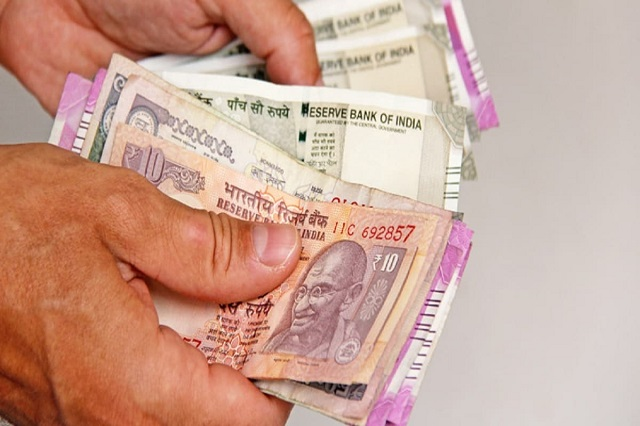# Rule of 72: Know when and how your money will double in PPF, SSY, KVP, NSC or Mutual FundsOn the basis of a short and simple rule, it can be estimated how much time the existing investment will double. It is also called Rule of 72. This rule gives an approximate idea of ​​doubling the money.

Everyone wants their capital to grow the fastest in the least amount of time. Doubling your money in any kind of investment depends on how long you invest and how much interest or return is earned on it. The more return or interest you get, the sooner your money will double. However, you can still find out about your current investment, how much time will this money double. For this, you just have to understand the rule of 72. So let’s know what this rule is all about.

Rule of 72 is a very simple formula, which very few people know about. Under this rule, you divide the interest received on your investment by ’72’. This gives you an idea that in how many days your money will double. The rule of 72 gives an approximate idea.

Suppose you have made a fixed deposit in a bank at an interest of 5% per annum. In this case, it will take about 14 years to double your money. Now you will say how it will take 14 years or how this figure was reached. For this, understand this small and very simple arithmetic.
Rule of 72
= 72/5
= 14.4 years

How much do you have to invest if you want to double your money? Now, after making a slight change in this formula, you can also find out how much you will have to invest in a fixed time that your money will double. Now let’s also know this.

If you want to double your money in just 3 years, then you should get a return of about 21 to 24 percent (72/3 years) every year. Similarly, if you want to double your money in 5 years, then for this you should get interest at the rate of at least 14.4 percent (72/5) every year. If you want to double the money in 10 years, then interest should be paid at the rate of around 7.2 percent every year.

>>What does this rule reveal about PPF, Sukanya Samriddhi Yojana (SSY), Kisana Vikas Patra (KVP), National Savings Certificate (NSC) and Mutual Funds? To calculate this, we have taken the current interest rate.

>> PPF is getting interest at the rate of 7.1 percent annually. In such a situation, after investing in PPF, it will take about 10 years (72 / 7.1 = 10.14) if the money doubles. But, there will be a condition for this that the interest rate will remain at 7.1 percent for the whole 10 years. Decrease or increase in interest rate will affect the duration.

>> Similarly, in Sukanya Samriddhi Yojana it will take about 9.4 years to double the money. Currently, under this scheme, interest is being received at the rate of 7.6 percent.

>> At the rate of 6.9 percent interest is being received on the Kisan Vikas Patra. Accordingly, putting money in KVP will double in 10.4 years.

>> National Savings Certificates are getting interest at the rate of 6.8 percent, according to which it will take 1.5 years to double the money.

>> At present the rate of returns of about 8.5% is given on short-term mutual funds and dynamic bonds. This is based on the past year. If you consider similar returns, then after investing in them, your money will double in about 8.4 years.